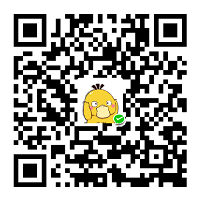1.1 Label控件不自动换行的问题

Duilib的Label控件在默认情况下是不支持文本换行的，比如在界面xml中有下面的Label控件定义：

<Label height="300" name="Label_Test" text="None" align="left" valign="top" font="0"/>

CLabelUI* temp_pLabel_Test = static_cast<CTextUI*>(m_PaintManager.FindControl(Str_Label_Test));
if (temp_pLabel_Test != nullptr)
{
std::stringstream ss;
for(int i=0;i<10;i++)
{
ss<<"a\n";
}
temp_pLabel_Test->SetText(ss.str().c_str());
}

1.2 问题的解决

<Label multiline="true" height="300" name="Label_Test" text="None" align="left" valign="top" font="0"/>

CLabelUI* temp_pLabel_Test = static_cast<CTextUI*>(m_PaintManager.FindControl(Str_Label_Test));
if (temp_pLabel_Test != nullptr)
{
temp_pLabel_Test->SetMultiLine(true);
}支付宝扫一扫微信扫一扫纵使晴明无雨色，入云深处亦沾衣。